﻿ Simple Calculator Flat Icon Stock Illustration - Illustration of cashier, clear: 144032159

# Simple calculator flat icon.

## Simple calculator flat icon, with simple and clear design, can be use for presentation and symbol for cashier

More similar stock illustrations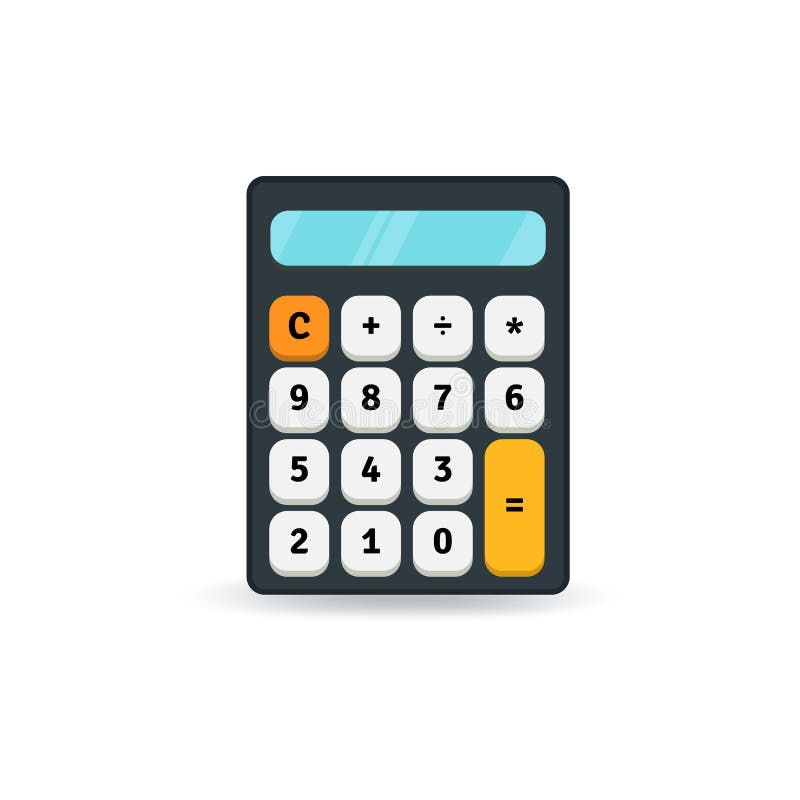Simple flat calculator icon isolated on white background. Design element.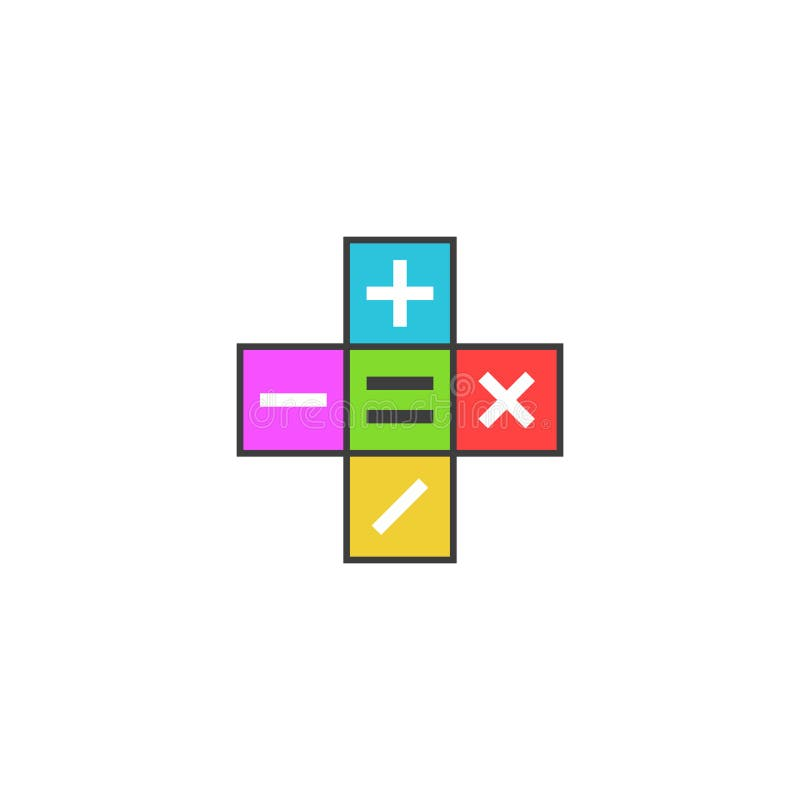Math calculator logo, Mathematical symbols plus, minus, subtract, multiply, equals icon on the colored tiles, simple modern flat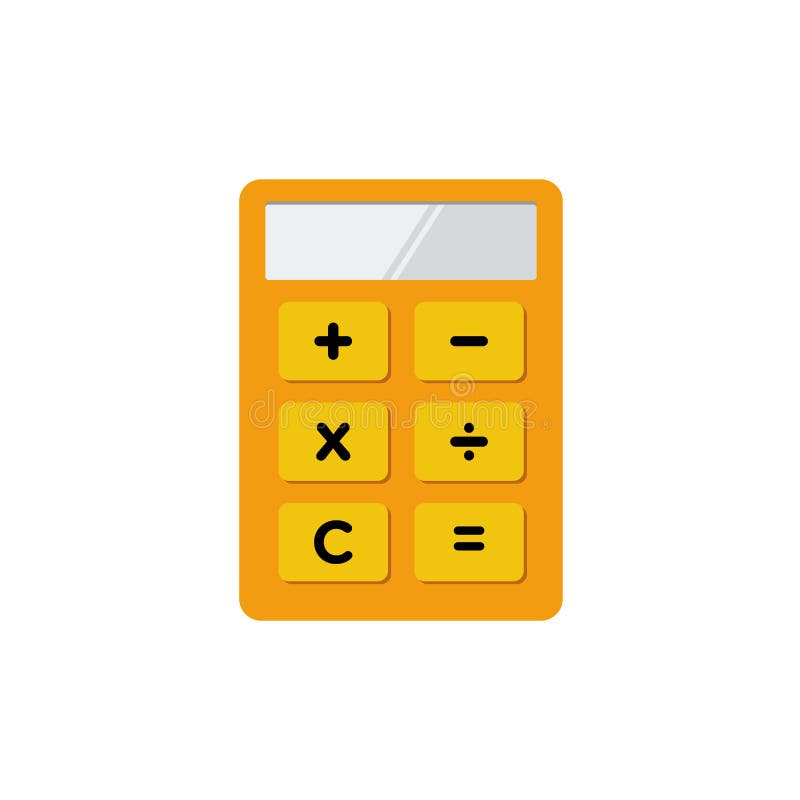Simple Flat minimalist Calculator symbol Icon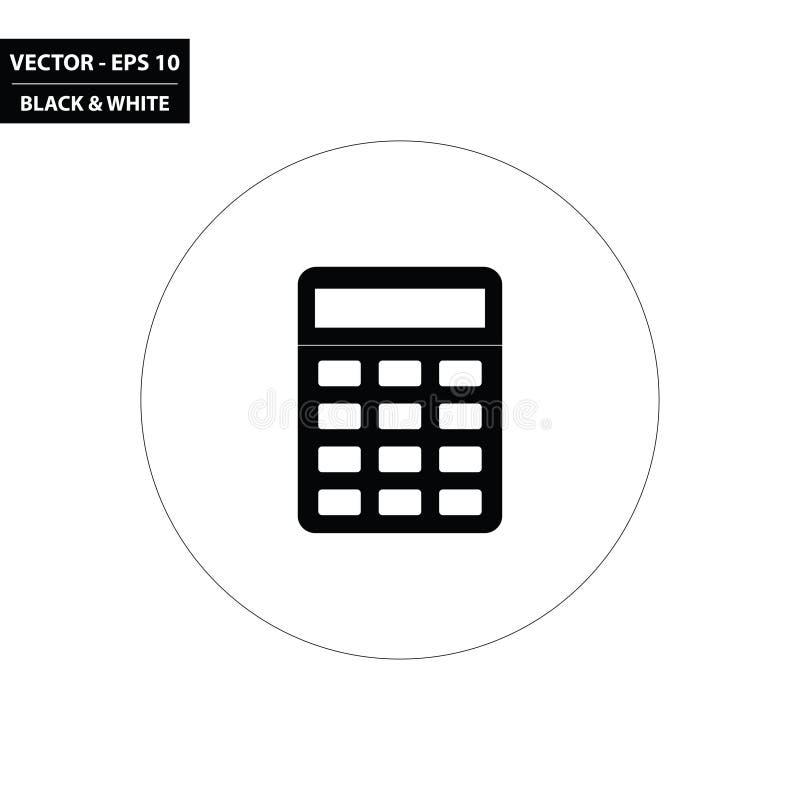Simple calculator black and white flat icon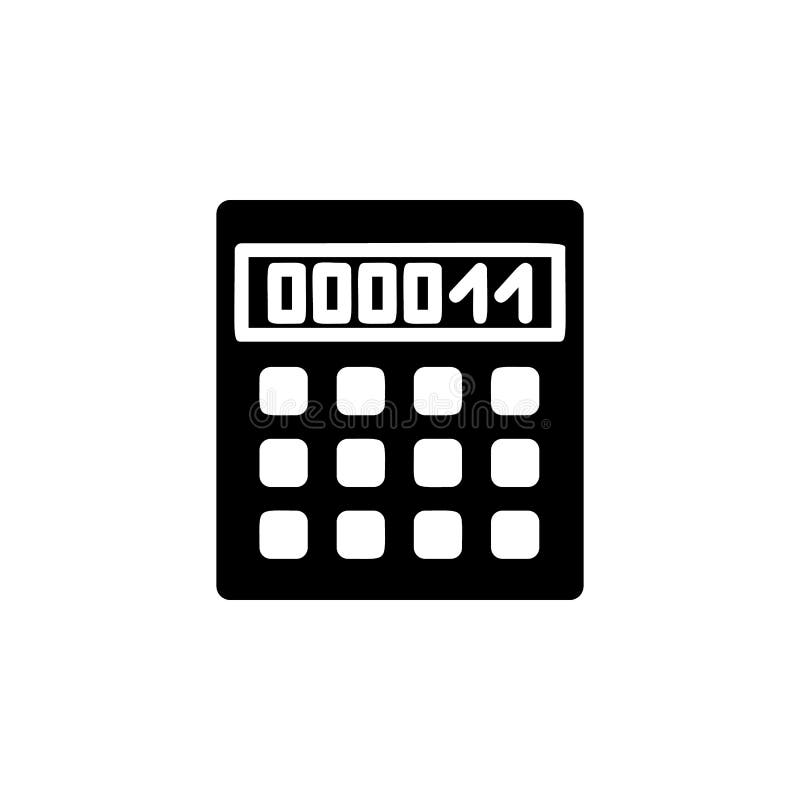Calculator icon. Simple glyph, flat vector of Web icons for UI and UX, website or mobile application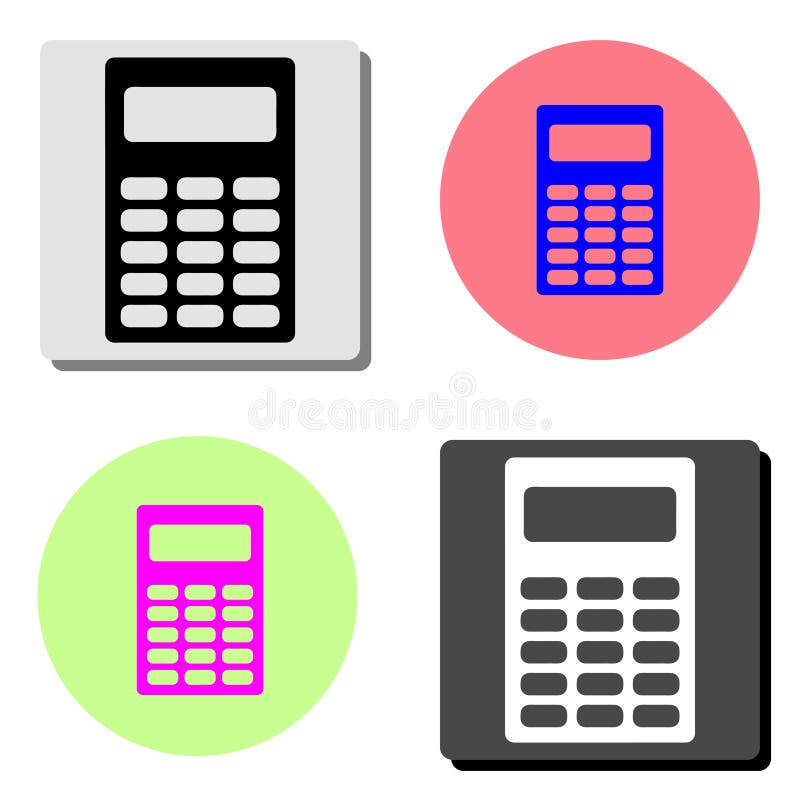Calculator. flat vector icon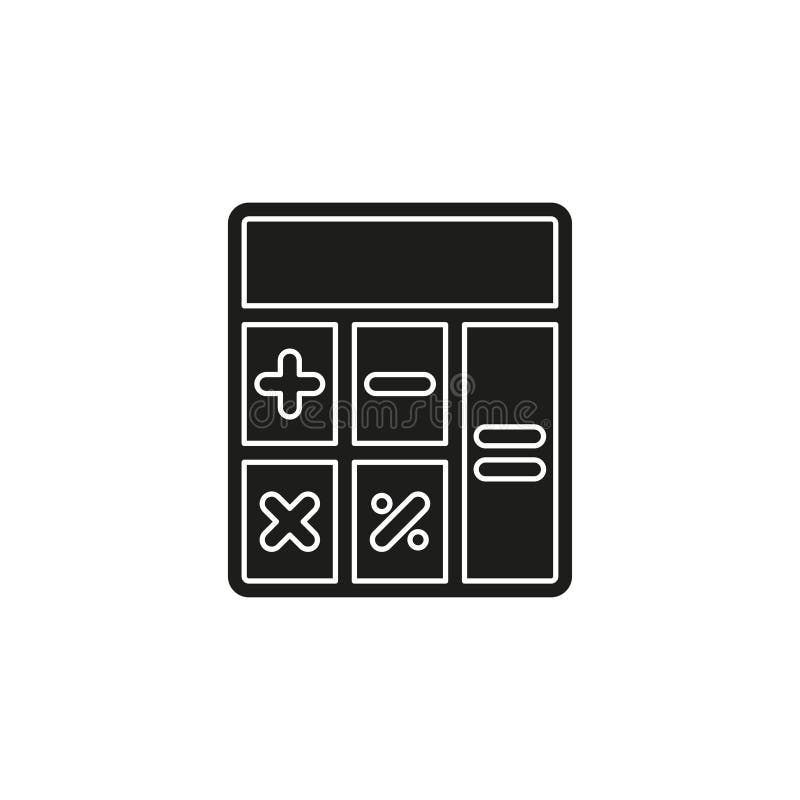Simple Calculator Vector Icon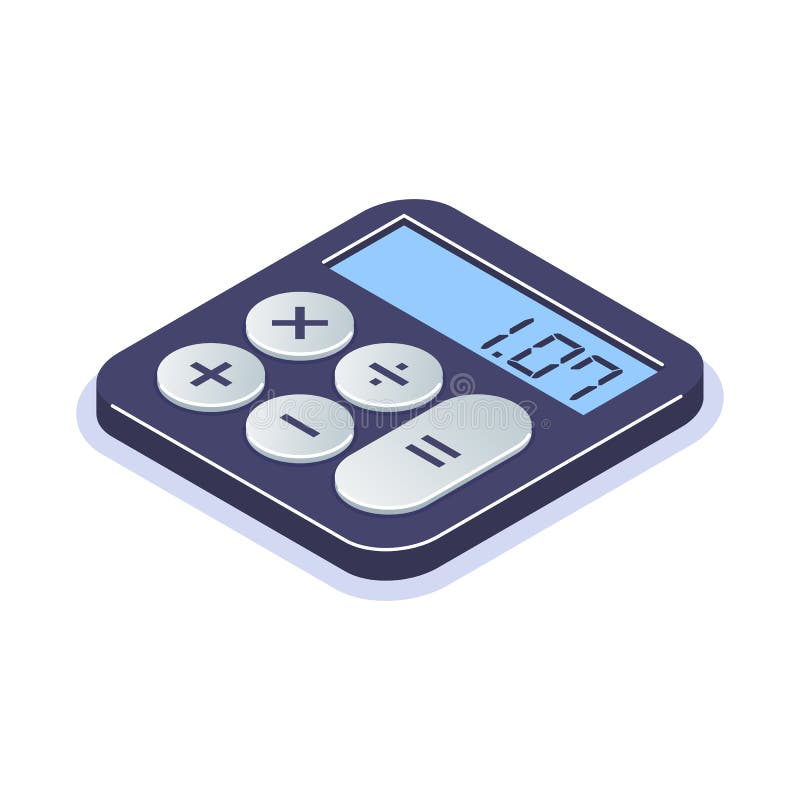Isometric flat calculator icon. Simple computing machine. Tool for arithmetic operations. Can use for web banner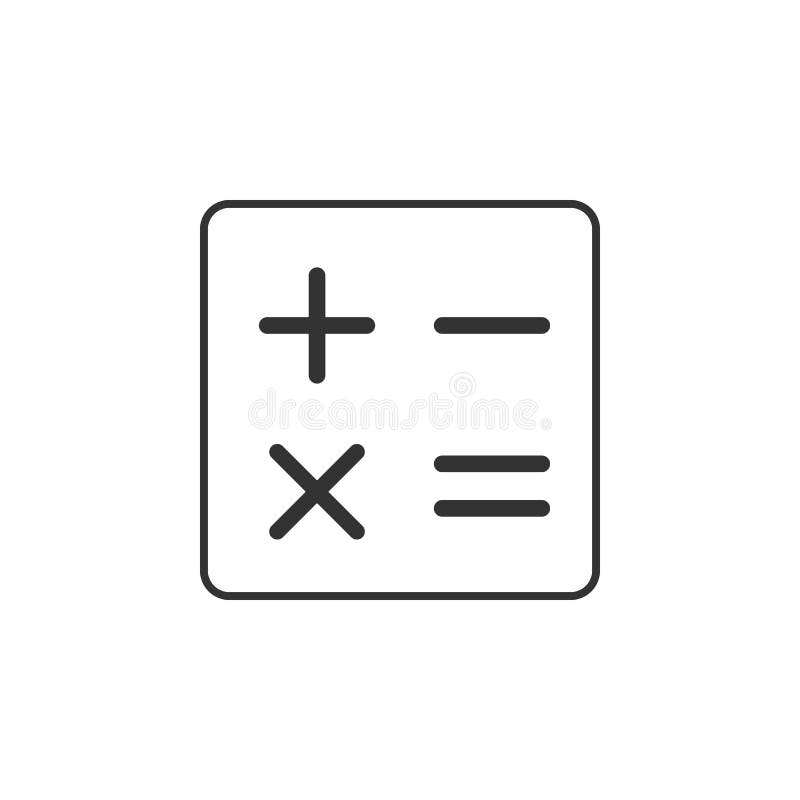Calculator, math line icon. Simple, modern flat vector illustration for mobile app, website or desktop app
Related categories